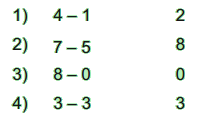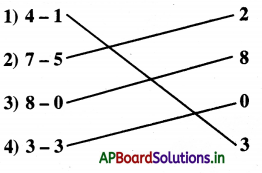# AP Board 1st Class Maths Solutions 3rd Lesson Subtraction

Andhra Pradesh AP Board 1st Class Maths Solutions 3rd Lesson Subtraction Textbook Exercise Questions and Answers.

## AP State Syllabus 1st Class Maths Solutions Chapter 3 Subtraction

Textbook Page No. 37Observe the above picture. Ask the children to give answers to the questions. Help them to identify the concept of subtraction.

Question 1.
What do you observe in the above picture ?
In the picture four children are playing and a boy is eating a mango. There are some birds, a mango tree and two rabbits eating carrots.

Question 2.
How many birds are there ?
6

Question 3.
How many birds are flying away ?
3Question 4.
How many birds are left on the tree ?
3

Question 5.
How many carrots are there ?
5

Question 6.
How many carrots are being eaten by rabbits ?
2

Question 7.
How many carrots are not eaten by rabbits ?
3

Textbook Page No. 39

How many are left? One is done for you.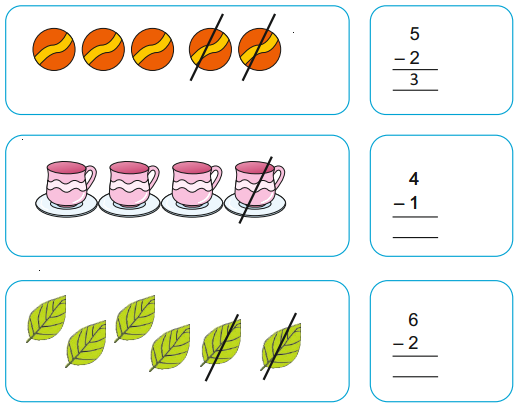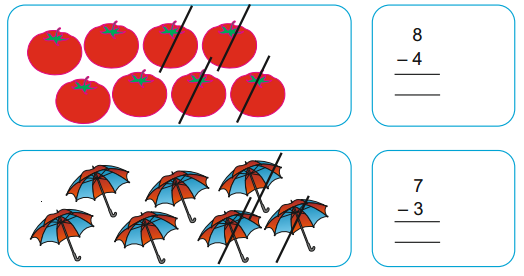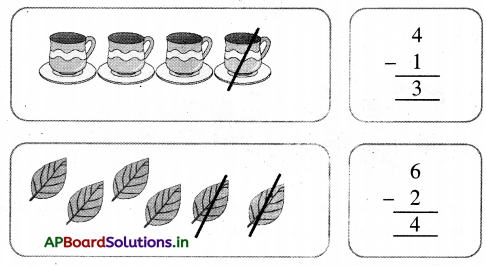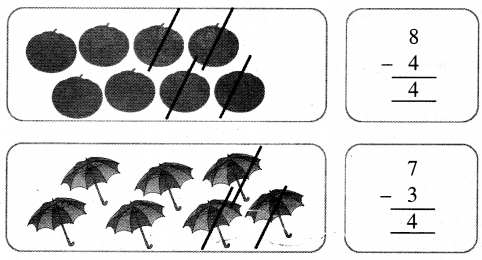Textbook Page No. 40

Vertical Subtraction:

The bigger number is written at the top. The smaller number which is to be subtracted is to be written below with a minus sign before it.
Eg. :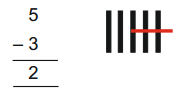Try this :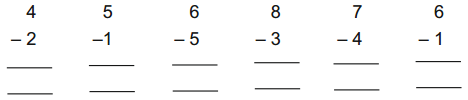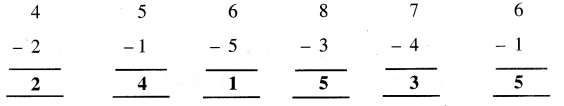Textbook Page No. 42, 43

Subtraction means taking away.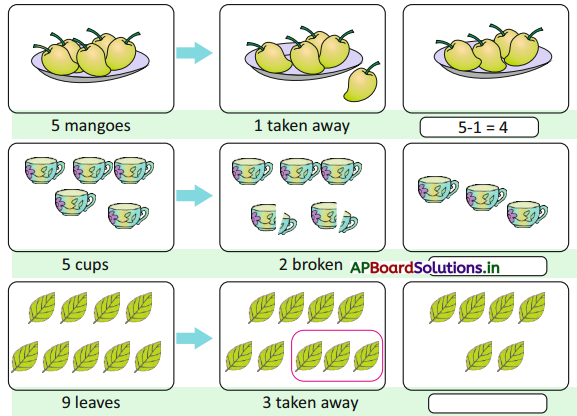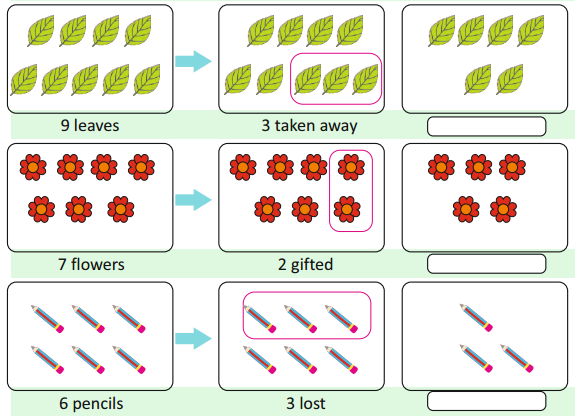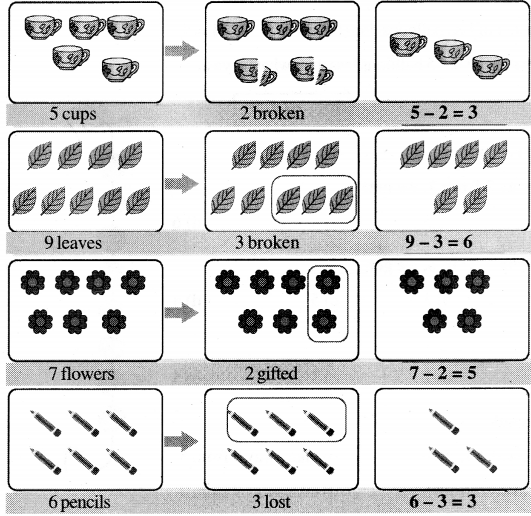Try this :

Question 1.
2 – 2 = _________
0

Question 2.
4 – 4 = __________
0

Question 3.
7 – 7 = __________
0

Question 4.
5 – 5 = ___________
0

Question 5.
8 – 8 = ____________
0Question 6.
9 – 9 = ___________
0

How many are ‘more’ or how many are ‘less’ ?

Question 1.
Girish has 3 pencils. How many more pencils does he need to make a bundle of 8 pencils ?
Total pencils needed = 8
Pencils Girish has = 3
The number of more pencils needed = 8 – 3 = 5
Girish needs 5 more pencils.

Question 2.
Seetal has 4 balloons. Priya has 9 balloons. How many less balloons does Seetal have ?
Priya’s balloons = 9
Seetal’shalloons = 4
The number of less balloons does he has = 9 – 4 = 5
Seetal has 5 balloons less than what Priya has.

Textbook Page No. 44

Exercise

Circle the correct option.Question 1.
Take away 2 from 7, we get
a) 7
b) 5
c) 4
d) 6
b) 5

Question 2.
Take away 4 from 6, we get
a) 2
b) 3
c) 5
d) 4
a) 2Question 3.
5 – 0 = __________
a) 0
b) 2
c) 3
d) 5
d) 5

Question 4.
9 – 5 means
a) take away 5 from 9
b) take away 9 from 5
c) take away 5 from 5
d) take away 9 from 9
a) take away 5 from 9

Question 5.
2 – ____________ = 2
a) 2
b) 0
c) 1
d) 3
b) 0

Question 6.
What is the mathematical representation of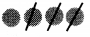a) 5 – 3
b) 4 – 2
c) 5 – 1
d) 4 – 3
d) 4 – 3Question 7.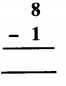a) 6
b) 5
c) 8
d) 7